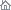Home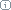About Us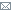ContactBlogWhat's NewProductsBuy NowDownloadsForumGeneXproTools Online Guide Learn how to use the 5 modeling platforms of GeneXproTools with the Online GuideLast update: February 19, 2014Log Odds Analysis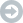Log Odds and Logistic Regression

The Log Odds Chart is central to the Logistic Regression Model. It’s with its aid that the slope and intercept of the Logistic Regression Model are calculated. And the algorithm is quite simple. As mentioned previously, it’s quantile-based and, in fact, just a few additional calculations are required to evaluate the regression parameters.

So, based on the Quantile Table, one first evaluates the odds ratio for all the bins (you have access to all the values on the Log Odds Table under Odds Ratio). Then the natural logarithm of this ratio (or the Log Odds) is evaluated (the Log Odds values are also shown on the Log Odds Table under Log Odds).

Note, however, that there might be a problem in the evaluation of the log odds if there are bins with zero positive cases. But this problem can be easily fixed with standard techniques. Although rare for large datasets, it can sometimes happen that some of the bins end up with zero positive cases in them. And this obviously results in a calculation error in the evaluation of the natural logarithm of the odds ratio. GeneXproTools handles this with a slight modification to the Laplace estimator to get what is called a complete Bayesian formulation with prior probabilities. In essence, this means that when a particular Quantile Table has bins with only negative cases, then we do the equivalent of priming all the bins with a very small amount of positive cases.

The formula GeneXproTools uses in the evaluation of the Positives Rate values pi for all the quantiles is the following: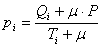where μ is the Laplace estimator that in GeneXproTools has the value of 0.01; Qi and Ti are, respectively, the number of Positive Cases and the number of Total Cases in bin i; and P is the Average Positive Rate of the whole dataset.

So, in the Log Odds Chart, the Log Odds values (adjusted or not with the Laplace strategy) are plotted on the Y-axis against the Model Output in the X-axis. And as for Quantile Regression, here there are also special rules to follow, depending on whether the predominant class is “1” or “0” and whether the model is normal or inverted. To be precise, the Log Odds are plotted against the Model Upper Boundaries if the predominant class is “1” and the model is normal, or the predominant class is “0” and the model is inverted; or against the Lower Boundaries if the predominant class is “1” and the model is inverted, or the predominant class is “0” and the model is normal.

Then a weighted linear regression is performed and the slope and intercept of the regression line are evaluated. And these are the parameters that will be used in the Logistic Regression Equation to evaluate the probabilities.

The regression line can be written as:where p is the probability of being “1”; x is the Model Output; and a and b are, respectively, the slope and intercept of the regression line. GeneXproTools draws the regression line and shows both the equation and the R-square in the Log Odds Chart.

And now solving the logistic equation above for p, gives: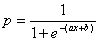which is the formula for evaluating the probabilities with the Logistic Regression Model. The probabilities estimated for each case are shown in the Logistic Fit Table.

Besides the slope and intercept of the Logistic Regression Model, another useful and widely used parameter is the exponent of the slope, usually represented by Exp(slope). It describes the proportionate rate at which the predicted odds ratio changes with each successive unit of x. GeneXproTools also shows this parameter both in the Log Odds Chart and in the companion Log Odds Stats Report.Logistic Fit Chart

The Logistic Fit Chart is a very useful graph that allows not only a quick visualization of how good the Logistic Fit is (the shape and steepness of the sigmoid curve are excellent indicators of the robustness and accuracy of the model), but also how the model outputs are distributed all over the model range.

The blue line (the sigmoid curve) on the graph is the logistic transformation of the model output x, using the slope a and intercept b calculated in the Log Odds Chart and is evaluated by the already familiar formula for the probability p:Since the proportion of Positive responses (1’s) and Negative responses (0’s) must add up to 1, both probabilities can be read on the vertical axis on the left. Thus, the probability of “1” is read directly on the vertical axis; and the probability of “0” is the distance from the line to the top of the graph, which is 1 minus the axis reading.

But there’s still more information on the Logistic Fit Chart. By plotting the dummy data points, which consist of up to 1000 randomly selected model scores paired with dummy random ordinates, one can clearly visualize how model scores are distributed. Are they all clumped together or are they finely distributed, which is the telltale sign of a good model? This is valuable information not only to guide the modeling process (not only in choosing model architecture and composition but also in the exploration of different fitness functions and class encodings that you can use to model your data), but also to sharpen one’s intuition and knowledge about the workings of learning evolutionary systems.

Indeed, browsing through the different models created in a run might prove both insightful and great fun. And you can do that easily as all the models in the Run History are accessible through the Model selector box in the Logistic Regression Window. Good models will generally allow for a good distribution of model outputs, resulting in a unique score for each different case. Bad models, though, will usually concentrate most of their responses around certain values and consequently are unable to distinguish between most cases. These are of course rough guidelines as the distribution of model outputs depends on multiple factors, including the type and spread of input variables and the complexity of the problem. For example, a simple problem may be exactly solved by a simple step function.

Below is shown a Gallery of Logistic Fit Charts typical of intermediate models generated during a GeneXproTools run. It was generated using the same models used to create the twin ROC Curve Gallery presented in the ROC Analysis section. The models were created for a risk assessment problem with a training dataset with 18,253 cases and using a small population of just 30 programs. The Classification Accuracy, the R-square, and the Area Under the ROC Curve (AUC) of each model, as well as the generation at which they were discovered, are also shown as illustration. From top to bottom, they are as follow:

 Generation 0, Accuracy = 65.33%, R-square = 0.0001, AUC = 0.5273 Generation 5, Accuracy = 66.03%, R-square = 0.0173, AUC = 0.5834 Generation 59, Accuracy = 66.92%, R-square = 0.0421, AUC = 0.6221 Generation 75, Accuracy = 68.99%, R-square = 0.1076, AUC = 0.7068 Generation 155, Accuracy = 69.93%, R-square = 0.1477, AUC = 0.7597 Generation 489, Accuracy = 74.15%, R-square = 0.2445, AUC = 0.7968Generation 0, Accuracy = 65.33%, R-square = 0.0001, AUC = 0.5273Generation 5, Accuracy = 66.03%, R-square = 0.0173, AUC = 0.5834Generation 59, Accuracy = 66.92%, R-square = 0.0421, AUC = 0.6221Generation 75, Accuracy = 68.99%, R-square = 0.1076, AUC = 0.7068Generation 155, Accuracy = 69.93%, R-square = 0.1477, AUC = 0.7597Generation 489, Accuracy = 74.15%, R-square = 0.2445, AUC = 0.7968

Besides its main goal, which is to estimate the probability of a response, the Logistic Regression Model can also be used to make categorical or binary predictions. From the logistic regression equation introduced in the previous section, we know that when a Positive event has the same probability of happening as a Negative one, the log odds term in the logistic regression equation becomes zero, giving: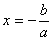where x is the model output at the Logistic Cutoff Point; and a and b are, respectively, the slope and the intercept of the regression line.

The Logistic Cutoff Point can be obviously used to evaluate a Confusion Matrix (in the Logistic Regression Window it is called Logistic Confusion Matrix to distinguish it from the ROC Confusion Matrix), in which model scores with Prob higher than or equal to 0.5 correspond to a Positive case and a Negative otherwise.

In the Logistic Fit Table, GeneXproTools shows the Most Likely Class, the Match, and Type values of the Logistic Confusion Matrix (you can see the graphical representation of the Logistic Confusion Matrix in the Confusion Matrix Tab). For easy visualization, the model output closest to the Logistic Cutoff Point is highlighted in light green in the Logistic Fit Table. Note that the exact value of the Logistic Cutoff Point is shown in the companion Logistic Fit Stats Report.

Related Tutorials:

Related Videos:

 Leave Feedback Please enter the number below using the combo boxes before sending your feedback. 3 8 4 0 1 2 3 4 5 6 7 8 9   0 1 2 3 4 5 6 7 8 9   0 1 2 3 4 5 6 7 8 9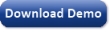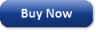Time Limited Trial

Try GeneXproTools for free for 30 days!

Released February 19, 2014

Last update: 5.0.3883New EntriesSubscribe to the GEP-List

 3 8 4 0 1 2 3 4 5 6 7 8 9   0 1 2 3 4 5 6 7 8 9   0 1 2 3 4 5 6 7 8 9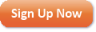Home | What's New | Products | Buy Now | Downloads | Quick Tour | Support | Contact Us | About Gepsoft | Sign UpForum | Blog | Videos | Tutorials | Server Knowledge Base | Logistic Regression Guide | Terms of Use | Privacy & Cookies